This book is archived and will be removed July 6, 2022. Please use the updated version.

Capital Budgeting Decisions

# 64 Evaluate the Payback and Accounting Rate of Return in Capital Investment Decisions

Many companies are presented with investment opportunities continuously and must sift through both viable and nonviable options to identify the best possible expenditure for business growth. The process to select the best option requires careful budgeting and analysis. In conducting their analysis, a company may use various evaluation methods with differing inputs and analysis features. These methods are often broken into two broad categories: (1) those that consider the time value of money, or the fact that a dollar today differs from a dollar in the future due to inflation and the ability to invest today’s money for future growth, and (2) those analysis methods that do not consider the time value of money. We will examine the non-time value methods first.

### Non-Time Value Methods

Non-time value methods do not compare the value of a dollar today to the value of a dollar in the future and are often used as screening tools. Two non-time value evaluative methods are the payback method and the accounting rate of return.

#### Fundamentals of the Payback Method

The payback method (PM) computes the length of time it takes a company to recover their initial investment. In other words, it calculates how long it will take until either the amount earned or the costs saved are equal to or greater than the costs of the project. This can be useful when a company is focused solely on retrieving their funds from a project investment as quickly as possible.

Businesses do not want their money tied up in capital assets that have limited liquidity. The longer money is unavailable, the less ability the company has to use these funds for other growth purposes. This extended length of time is also a concern because it produces a riskier opportunity. Therefore, a company would like to get their money returned to them as quickly as possible. One way to focus on this is to consider the payback period when making a capital budget decision. The payback method is limited in that it only considers the time frame to recoup an investment based on expected annual cash flows, and it doesn’t consider the effects of the time value of money.

The payback period is calculated when there are even or uneven annual cash flows. Cash flow is money coming into or out of the company as a result of a business activity. A cash inflow can be money received or cost savings from a capital investment. A cash outflow can be money paid or increased cost expenditures from capital investment. Cash flow will estimate the ability of the company to pay long-term debt, its liquidity, and its ability to grow. Cash flows appear on the statement of cash flows. Cash flows are different than net income. Net income will represent all company activities affecting revenues and expenses regardless of the occurrence of a cash transaction and will appear on the income statement.

A company will estimate the future cash inflows and outflows to be generated by the capital investment. It’s important to remember that the cash inflows can be caused by an increase in cash receipts or by a reduction in cash expenditures. For example, if a new piece of equipment would reduce the production costs for a company from ?120,000 a year to ?80,000 a year, we would consider this is a ?40,000 cash inflow. While the company does not actually receive the ?40,000 in cash, it does save ?40,000 in operating costs giving it a positive cash inflow of ?40,000.

Cash flow can also be generated through increased production volume. For example, a company purchases a new building costing ?100,000 that will allow them to house more space for production. This new space allows them to produce more product to sell, which increases cash sales by ?300,000. The ?300,000 is a new cash inflow.

The difference between cash inflows and cash outflows is the net cash inflow or outflow, depending on which cash flow is larger.Annual net cash flows are then related to the initial investment to determine a payback period in years. When the expected net annual cash flow is an even amount each period, payback can be computed as follows:The result is the number of years it will take to recover the cash made in the original investment. For example, a printing company is considering a printer with an initial investment cost of ?150,000. They expect an annual net cash flow of ?20,000. The payback period is

$$\text{Payback Period}=\frac{?150,000}{?20,000}=7.5\phantom{\rule{0.2em}{0ex}}\text{years}$$

The initial investment cost of ?150,000 is divided by the annual cash flow of ?20,000 to compute an expected payback period of 7.5 years. Depending on the company’s payback period requirements for this type of investment, they may pass this option through the screening process to be considered in a preference decision. For example, the company might require a payback period of 5 years. Since 7.5 years is greater than 5 years, the company would probably not consider moving this alternative to a preference decision. If the company required a payback period of 9 years, the company would consider moving this alternative to a preference decision, since the number of years is less than the requirement.

When net annual cash flows are uneven over the years, as opposed to even as in the previous example, the company requires a more detailed calculation to determine payback. Uneven cash flows occur when different amounts are returned each year. In the previous printing company example, the initial investment cost was ?150,000 and even cash flows were ?20,000 per year. However, in most examples, organizations experience uneven cash flows in a multiple-year ownership period. For example, an uneven cash flow distribution might be a return of ?10,000 in year one, ?20,000 in years two and three, ?15,000 in years four and five, and ?20,000 in year six and beyond.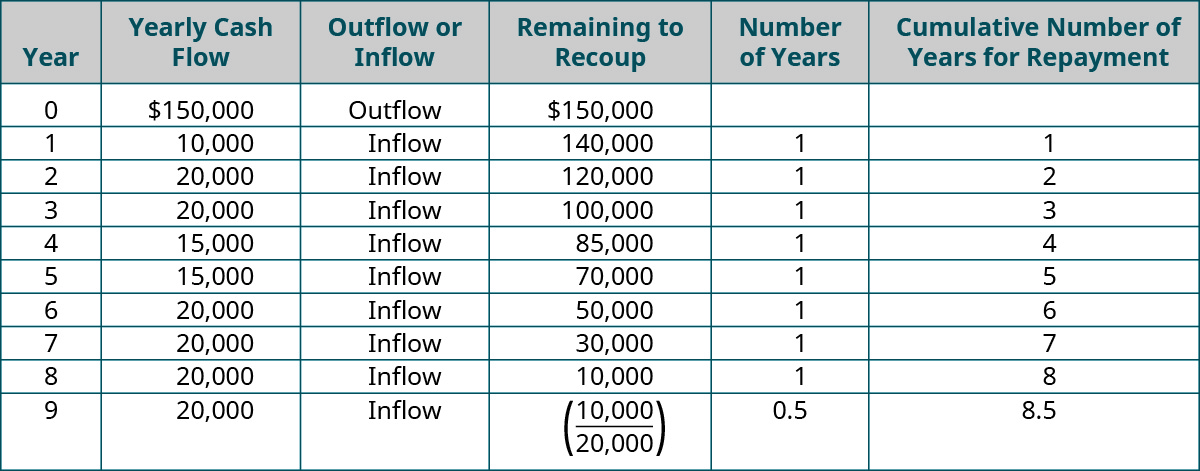In this case, then, the payback period is 8.5 years.

In a second example of the payback period for uneven cash flows, consider a company that will need to determine the net cash flow for each period and figure out the point at which cash flows equal or exceed the initial investment. This could arise in the middle of a year, prompting a calculation to determine the partial year payback.The company would add the partial year payback to the prior years’ payback to get the payback period for uneven cash flows. For example, a company may make an initial investment of ?40,000 and receive net cash flows of ?10,000 in years one and two, ?5,000 in year three and four, and ?7,500 for years five and beyond.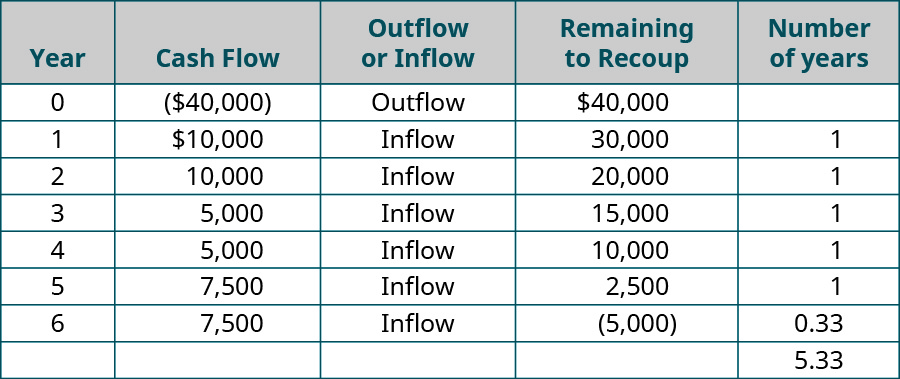We know that somewhere between years 5 and 6, the company recovers the money. In years one and two they recovered a total of ?20,000 (10,000 + 10,000), in years three and four they recovered and additional ?10,000 (5,000 + 5,000), and in year five they recovered ?7,500, for a total through year five of ?37,500. This left an outstanding balance after year five of ?2,500 (40,000 – 37,500) to fully recover the costs of the investment. In year six, they had a cash flow of ?7,500. This is more than they needed to recoup their initial investment. To get a more specific calculation, we need to compute the partial year’s payback.

$$\text{Partial Year Payback}=\frac{?2,500}{?7,500}=0.33\phantom{\rule{0.2em}{0ex}}\text{years (rounded)}$$

Therefore, the total payback period is 5.33 years (5 years + 0.33 years).

#### Demonstration of the Payback Method

For illustration, consider Baby Goods Manufacturing (BGM), a large manufacturing company specializing in the production of various baby products sold to retailers. BGM is considering investment in a new metal press machine. The payback period is calculated as follows:

$$\text{Payback Period}=\frac{?50,000}{?15,000}=3.33\phantom{\rule{0.2em}{0ex}}\text{years}$$

We divide the initial investment of ?50,000 by the annual inflow of ?15,000 to arrive at a payback period of 3.33 years. Assume that BGM will not allow a payback period of more than 7 years for this type of investment. Since this computed payback period meets their initial screening requirement, they can pass this investment opportunity on to a preference decision level. If BGM had an expected or maximum allowable payback period of 2 years, the same investment would not have passed their screening requirement and would be dropped from consideration.

To illustrate the concept of uneven cash flows, let’s assume BGM shows the following expected net cash flows instead. Recall that that the initial investment in the metal press machine is ?50,000.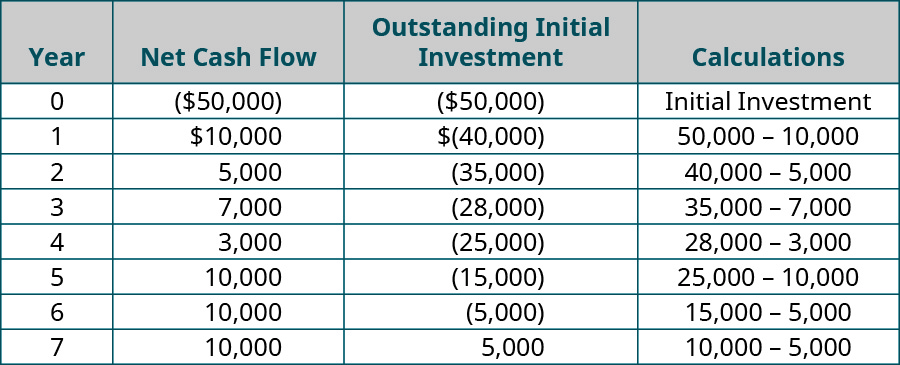Between years 6 and 7, the initial investment outstanding balance is recovered. To determine the more specific payback period, we calculate the partial year payback.

$$\text{Payback Period}=\frac{?5,000}{?10,000}=0.5\phantom{\rule{0.2em}{0ex}}\text{years}$$

The total payback period is 6.5 years (6 years + 0.5 years).

Capital Investment

You are the accountant at a large firm looking to make a capital investment in a future project. Your company is considering two project investments. Project A’s payback period is 3 years, and Project B’s payback period is 5.5 years.

Your company requires a payback period of no more than 5 years on such projects. Which project should they further consider? Why? Is there an argument that can be made to advance either project or neither project? Why? What other factors might be necessary to make that decision?

#### Fundamentals of the Accounting Rate of Return Method

The accounting rate of return (ARR) computes the return on investment considering changes to net income. It shows how much extra income the company could expect if it undertakes the proposed project. Unlike the payback method, ARR compares income to the initial investment rather than cash flows. This method is useful because it reviews revenues, cost savings, and expenses associated with the investment and, in some cases, can provide a more complete picture of the impact, rather than focusing solely on the cash flows produced. However, ARR is limited in that it does not consider the value of money over time, similar to the payback method.

The accounting rate of return is computed as follows:Incremental revenues represent the increase to revenue if the investment is made, as opposed to if the investment is rejected. The increase to revenues includes any cost savings that occur because of the project. Incremental expenses show the change to expenses if the project is accepted as opposed to maintaining the current conditions. Incremental expenses also include depreciation of the acquired asset. The difference between incremental revenues and incremental expenses is called the incremental net income. The initial investment is the original amount invested in the project; however, any salvage (residual) value for the capital asset needs to be subtracted from the initial investment before obtaining ARR.

The concept of salvage value was addressed in Long-Term Assets. Basically, it is the anticipated future fair market value (FMV) of an asset when it is to be sold or used as a trade-in for a replacement asset. For example, assume that you bought a commercial printer for ?40,000 five years ago with an anticipated salvage value of ?8,000, and you are now considering replacing it. Assume that as of the date of replacement after the five-year holding period, the old printer has an FMV of ?8,000. If the new printer has a purchase price of ?45,000 and the seller is going to take the old printer as a trade-in, then you would owe ?37,000 for the new printer. If the printer had been sold for ?8,000, instead being used as a trade-in, the ?8,000 could have been used as a down payment, and the company would still owe ?37,000. This amount is the price of ?45,000 minus the FMV value of ?8,000.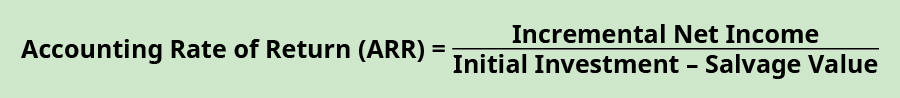There is one more point to make with this example. The fair market value is not the same as the book value. The book value is the original cost less the accumulated depreciation that has been taken. For example, if you buy a long-term asset for ?60,000 and the accumulated depreciation that you have taken is ?42,000, then the asset’s book value would be ?18,000. The fair market value could be more, less, or the same as the book value.

For example, a piano manufacturer is considering investment in a new tuning machine. The initial investment will cost ?300,000. Incremental revenues, including cost savings, are ?200,000, and incremental expenses, including depreciation, are ?125,000. ARR is computed as:

$$\text{ARR}=\frac{\left(\text{?}200,000-\text{?}125,000\right)}{\text{?}300,000}=0.25\phantom{\rule{0.2em}{0ex}}\text{or}\phantom{\rule{0.2em}{0ex}}25%$$

This outcome means the company can expect an increase of 25% to net income, or an extra 25 cents on each dollar, if they make the investment. The company will have a minimum expected return that this project will need to meet or exceed before further consideration is given. ARR, like payback method, should not be used as the sole determining factor to invest in a capital asset. Also, note that the ARR calculation does not consider uneven annual income growth, or other depreciation methods besides straight-line depreciation.

#### Demonstration of the Accounting Rate of Return Method

Returning to the BGM example, the company is still considering the metal press machine because it passed the payback period method of less than 7 years. BGM has a set rate of return of 25% expected for the metal press machine investment. The company expects incremental revenues of ?22,000 and incremental expenses of ?12,000. Remember that the initial investment cost is ?50,000. BGM computes ARR as follows:

$$\text{ARR}=\frac{\left(\text{?}20,000-\text{?}5,000\right)}{\text{?}50,000}=0.3\phantom{\rule{0.2em}{0ex}}\text{or}\phantom{\rule{0.2em}{0ex}}30%$$

The ARR in this situation is 30%, exceeding the required hurdle rate of 25%. A hurdle rate is the minimum required rate of return on an investment to consider an alternative for further evaluation. In this case, BGM would move this investment option to a preference decision level. If we were to add a salvage value of ?5,000 into the situation, the computation would change as follows:

$$\text{ARR}=\frac{\left(\text{?}20,000-\text{?}5,000\right)}{\text{?}50,000-\text{?}5,000\right)}=0.33\phantom{\rule{0.2em}{0ex}}\text{or}\phantom{\rule{0.2em}{0ex}}33%\phantom{\rule{0.2em}{0ex}}\text{(rounded)}.$$

The ARR still exceeds the hurdle rate of 25%, so BGM would still forward the investment opportunity for further consideration. Let’s say BGM changes their required return rate to 35%. In both cases, the project ARR would be less than the required rate, so BGM would not further consider either investment.

Analyzing Hurdle Rate

Turner Printing is looking to invest in a printer, which costs ?60,000. Turner expects a 15% rate of return on this printer investment. The company expects incremental revenues of ?30,000 and incremental expenses of ?15,000. There is no salvage value for the printer. What is the accounting rate of return (ARR) for this printer? Did it meet the hurdle rate of 15%?

Solution

ARR is 25% calculated as (?30,000 – ?15,000) / ?60,000. 25% exceeds the hurdle rate of 15%, so the company would consider moving this alternative to a preference decision.

Both the payback period and the accounting rate of return are useful analytical tools in certain situations, particularly when used in conjunction with other evaluative techniques. In certain situations, the non-time value methods can provide relevant and useful information. However, when considering projects with long lives and significant costs to initiate, there are more advanced models that can be used. These models are typically based on time value of money principles, the basics of which are explained here.

Analyzing Investments

Your company is considering making an investment in equipment that will cost ?240,000. The equipment is expected to generate annual cash flows of ?60,000, provide incremental cash revenues of ?200,000, and provide incremental cash expenses of ?140,000 annually. Depreciation expense is included in the ?140,000 incremental expense.

Calculate the payback period and the accounting rate of return.

Solution

$$\begin{array}{ccc}\hfill \text{Payback Period}& =\hfill & \frac{?240,000}{60,000}=4\phantom{\rule{0.2em}{0ex}}\text{years}\hfill \\ \hfill \text{ARR}& =\hfill & \frac{\left(?200,000–?140,000\right)}{240,000}=25%\hfill \end{array}$$

### Key Concepts and Summary

• The payback method determines how long it will take a company to recoup their investment. Annual cash flows are compared to the initial investment but the time value of money is not considered and cashflows beyond the payback period are ignored.
• The accounting rate of return considers incremental net income as it compares to the initial investment. Time value of money is not considered with this method.
• Incremental net income determines the net income expected if the company accepts the investment opportunity, as opposed to not investing. Incremental net income is the difference between incremental revenues and incremental expenses.

(Figure)What is the payback method used to determine?

It is used to determine the length of time needed for a long-term project to recapture or pay back the initial investment in the project.

(Figure)What are one advantage and one disadvantage of the accounting rate of return method?

Advantage: The ARR compares income to the initial investment rather than to cash flows; thus, incremental revenues, cost savings, and incremental expenses associated with the investment are reviewed and provide a more complete picture than payback, which uses cash flows. Disadvantage: ARR is limited in that it does not consider the value of a dollar over time.

(Figure)What is the equation to calculate the payback period?

(Figure)What is the equation to calculate the accounting rate of return?

Accounting Rate of Return = (Incremental revenues – Incremental expenses) ÷ Initial Investment

(Figure)If a copy center is considering the purchase of a new copy machine with an initial investment cost of ?150,000 and the center expects an annual net cash flow of ?20,000 per year, what is the payback period?

(Figure)Assume a company is going to make an investment of ?450,000 in a machine and the following are the cash flows that two different products would bring in years one through four. Which of the two options would you choose based on the payback method?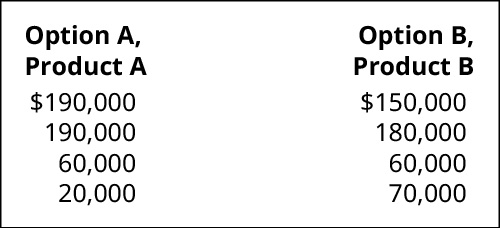(Figure)If a garden center is considering the purchase of a new tractor with an initial investment cost of ?120,000, and the center expects a return of ?30,000 in year one, ?20,000 in years two and three, ?15,000 in years four and five, and ?10,000 in year six and beyond, what is the payback period?

(Figure)The management of Kawneer North America is considering investing in a new facility and the following cash flows are expected to result from the investment: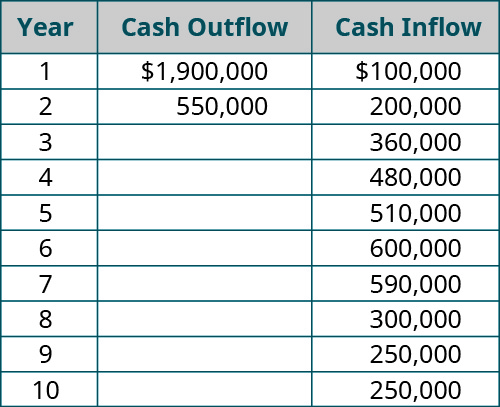1. What is the payback period of this uneven cash flow?
2. Does your answer change if year 10’s cash inflow changes to ?500,000?

(Figure)A mini-mart needs a new freezer and the initial investment will cost ?300,000. Incremental revenues, including cost savings, are ?200,000, and incremental expenses, including depreciation, are ?125,000. There is no salvage value. What is the accounting rate of return (ARR)?

(Figure)A restaurant is considering the purchase of new tables and chairs for their dining room with an initial investment cost of ?515,000, and the restaurant expects an annual net cash flow of ?103,000 per year. What is the payback period?

(Figure)Assume a company is going to make an investment in a machine of ?825,000 and the following are the cash flows that two different products would bring. Which of the two options would you choose based on the payback method?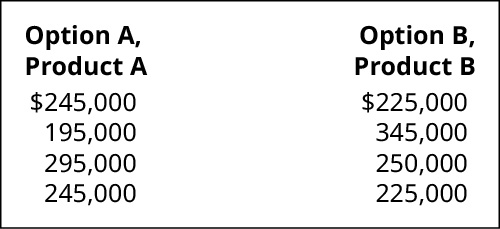(Figure)A grocery store is considering the purchase of a new refrigeration unit with an initial investment of ?412,000, and the store expects a return of ?100,000 in year one, ?72,000 in years two and three, ?65,000 in years four and five, and ?38,000 in year six and beyond, what is the payback period?

(Figure)The management of Ryland International is considering investing in a new facility and the following cash flows are expected to result from the investment: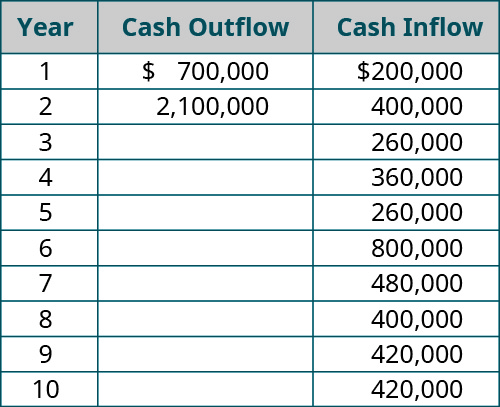1. What is the payback period of this uneven cash flow?
2. Does your answer change if year 6’s cash inflow changes to ?920,000?

(Figure)An auto repair company needs a new machine that will check for defective sensors. The machine has an initial investment of ?224,000. Incremental revenues, including cost savings, are ?120,000, and incremental expenses, including depreciation, are ?50,000. There is no salvage value. What is the accounting rate of return (ARR)?

(Figure)Your company is planning to purchase a new log splitter for its lawn and garden business. The new splitter has an initial investment of ?180,000. It is expected to generate ?25,000 of annual cash flows, provide incremental cash revenues of ?150,000, and incur incremental cash expenses of ?100,000 annually.

What is the payback period and accounting rate of return (ARR)?

(Figure)Jasmine Manufacturing is considering a project that will require an initial investment of ?52,000 and is expected to generate future cash flows of ?10,000 for years 1 through 3, ?8,000 for years 4 and 5, and ?2,000 for years 6 through 10. What is the payback period for this project?

(Figure)A bookstore is planning to purchase an automated inventory/remote marketing system, which includes an upgrade to a more sophisticated cash register system. The package has an initial investment cost of ?360,000. It is expected to generate ?144,000 of annual cash flows, reduce costs and provide incremental cash revenues of ?326,000, and incur incremental cash expenses of ?200,000 annually.

What is the payback period and accounting rate of return (ARR)?

(Figure)Markoff Products is considering two competing projects, but only one will be selected. Project A requires an initial investment of ?42,000 and is expected to generate future cash flows of ?6,000 for each of the next 50 years. Project B requires an initial investment of ?210,000 and will generate ?30,000 for each of the next 10 years. If Markoff requires a payback of 8 years or less, which project should it select based on payback periods?

### Glossary

accounting rate of return (ARR)
return on investment considering changes to net income
cash flow
cash receipts and cash disbursements as a result of business activity
cash inflow
money received or cost savings from a capital investment
cash outflow
money paid or increased cost expenditures from capital investment
hurdle rate
minimum required rate of return on an investment to consider an alternative for further evaluation
non-time value methods
analysis that does not consider the comparison value of a dollar today to a dollar in the future
payback method (PM)
calculation of the length of time it takes a company to recoup their initial investment